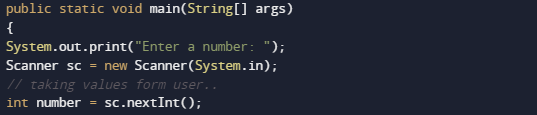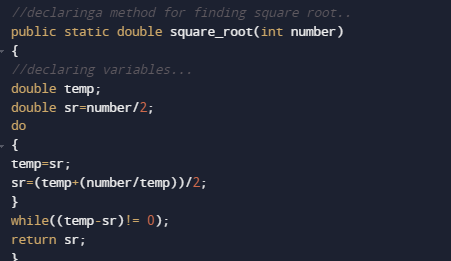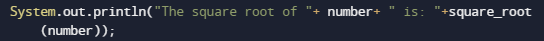# Java Program to Find Square Root of a Number

In this tutorial you will learn about the Java Program to Find Square Root of a Number and its application with practical example.

In this tutorial, we will learn to create a Java program to Find square Root of a number using Java programming.

## Prerequisites

Before starting with this tutorial we assume that you are best aware of the following Java programming topics:

• Java Operators.
• Basic Input and Output
• Class and Object.
• Basic Java programming.
• if-else statements.
• Switch case.
• Nested if-else Statements.
• Java Scanner class.

## What Is Square root?

Method of Finding “Square Root” is that numbers are multiplying by itself Twice “6 * 6= 36”. Where a square root of a number on getting multiplied by itself gives the original √36 = 6.
A square root of a number a is a number b such that =a.

## Java Program to Find Square Root of a Number

In this our program we will create a program to find “Square Root” of a given number . We would first declared and initialized the required variables. Next, we would prompt user to input value.Later in the program we will find square root.

## Output

Square RootIn the above program, we have first declared and initialized a set variables required in the program.

• number = it will hold  given number.
• temp= it will hold temporary value of a number
• sr= it will square root of a number.We going to use the following formula to find the square root of a number.in our program we have created a method square_root(number), in this method we have written a equation for finding the square root of a number.and for equation we have used do while loop.function returns the square root of a given number to main methodWhere we will print the required square root of a given number as shown in image.In this tutorial we have learn about the Java Program to Find Square Root of a Number and its application with practical example. I hope you will like this tutorial.